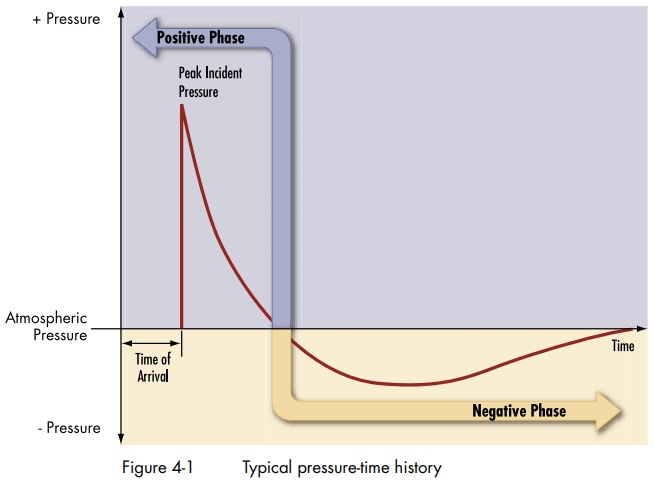# The Physics of Explosions Tossing Girders - Overpressure and Impulse

Discussion in '9/11' started by Mick West, Dec 10, 2018.

Tags:

The idea that explosion in the World Trade Center tossed multi-ton girders hundreds of feet has always been something that seems ridiculous, and is generally dismissed as such. But what's the actual physics?

https://www.fema.gov/media-library-data/20130726-1455-20490-7465/fema426_ch4.pdfThis diagram is a typical way of describing the effects of an explosion. It describes the air pressure changes of an explosion. It's this pressure that pushes things.

Pressure is measured in pounds per square inch, which seems quite simple, if a wall section or girder has a cross section of, say 50 feet, and the peak incident overpressure is 50 PSI then the force exerted is 250 * 12 * 12 * 50 = 1,800,000 pounds.

So how fast will a girder go if you apply 1,800,000 pounds of force to it? It depends how long. Explosions are very short events, a fraction of a second, the pressure also decays rapidly.

So what we actually want is the impulse, the integral of force over time, or put more simply the area of that triangle. It's essentially the average force multiplied by time.

The units that we use to measure impulse are the same as we use to measure momentum, so in standard (metric) units it's mass * velocity, or mass * movement / time, or kg.m/s (kilograms times meters per second).

So, we can work backward here, if we know a girder has to be accelerated to around 60 mph (around 25 m/s), and we know it weights, say, 10 tonnes (10,000 kg), then we know that the impulse (the area of that triangle) was 10000 * 25 = 250,000 kg.m/s

(to be continued)

•Informative x 1